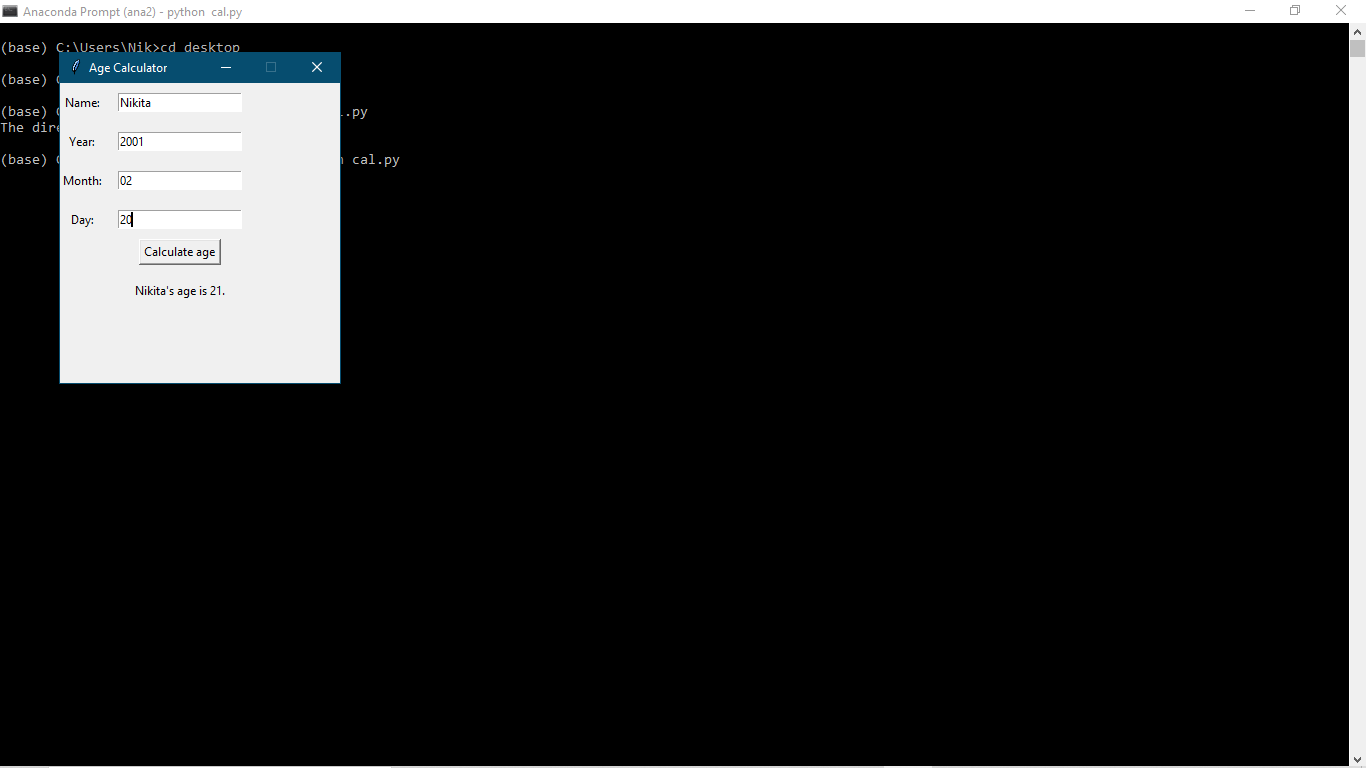Age Calculator GUI(Python projects ideas with source code series):

Another python project for beginners.

As we all know the best learning is by doing. So I have started this python projects for beginners series with source code for beginners and intermediate level python developers.

In this project, I have made a graphical calculator using python, which collects all the information about a person by the graphical interface and calculates their age.

The calculator asks person's name, and birthdate(year and month) and calculates age according to that information. This simple python project will help beginners to build confidence in their python coding career.

So Let’s Get Started:

To start I’m going to create a new python file, Let’s name it cal.py(The extension for the python file is .py) and import two libraries into our code. The first one is the Tkinter library. Python Tkinter is the standard Graphical User Interface (GUI) that is supported in Python. When Tkinter is used alongside Python, it results in the creation of GUI very conveniently and quite fast. Then, we need the DateTime library to work with dates. To import library.

pip install tkinter

pip install datetime

``````
# import libraries
from tkinter import *
from datetime import date

# initialized window
root = Tk()
root.geometry('280x300')
root.resizable(0, 0)
root.title('Age Calculator')
statement = Label(root)

# defining the function for calculating age

def ageCalc():
global statement
statement.destroy()
today = date.today()
birthDate = date(int(yearEntry.get()), int(
monthEntry.get()), int(dayEntry.get()))
age = today.year - birthDate.year
if today.month < birthDate.month or today.month == birthDate.month and today.day < birthDate.day:
age -= 1
statement = Label(text=f"{nameValue.get()}'s age is {age}.")

# creating a label for person's name to display
l1 = Label(text="Name: ")
l1.grid(row=1, column=0)
nameValue = StringVar()

# creating a entry box for input
nameEntry = Entry(root, textvariable=nameValue)

# label for year in which user was born
l2 = Label(text="Year: ")
l2.grid(row=2, column=0)
yearValue = StringVar()
yearEntry = Entry(root, textvariable=yearValue)

# label for month in which user was born
l3 = Label(text="Month: ")
l3.grid(row=3, column=0)
monthValue = StringVar()
monthEntry = Entry(root, textvariable=monthValue)

# label for day/date on which user was born
l4 = Label(text="Day: ")
l4.grid(row=4, column=0)
dayValue = StringVar()
dayEntry = Entry(root, textvariable=dayValue)

# create a button for calculating age
button = Button(text="Calculate age", command=ageCalc)
button.grid(row=5, column=1)

# infinite loop to run program
root.mainloop()

```
```
Let’s dive In step by step on the code :

First import all libraries

from tkinter import *

from datetime import date

#After that to initialized window

root = Tk()

root.geometry('280x300')

root.resizable(0, 0)

root.title('Age Calculator')

statement = Label(root)

#Then the main part is  defining the function for calculating age

def ageCalc():

global statement

statement.destroy()

today = date.today()

birthDate = date(int(yearEntry.get()), int(

monthEntry.get()), int(dayEntry.get()))

age = today.year - birthDate.year

if today.month < birthDate.month or today.month == birthDate.month and today.day < birthDate.day:

age -= 1

statement = Label(text=f"{nameValue.get()}'s age is {age}.")

#This creating a label for person's name to display

l1 = Label(text="Name: ")

l1.grid(row=1, column=0)

nameValue = StringVar()

# The second part is to creating a entry box for input

nameEntry = Entry(root, textvariable=nameValue)

#Using this we create label for year in which user was born

l2 = Label(text="Year: ")

l2.grid(row=2, column=0)

yearValue = StringVar()

yearEntry = Entry(root, textvariable=yearValue)

#Create label for month in which user was born

l3 = Label(text="Month: ")

l3.grid(row=3, column=0)

monthValue = StringVar()

monthEntry = Entry(root, textvariable=monthValue)

# label for day/date on which user was born

l4 = Label(text="Day: ")

l4.grid(row=4, column=0)

dayValue = StringVar()

dayEntry = Entry(root, textvariable=dayValue)

# create a button for calculating age this is important part

button = Button(text="Calculate age", command=ageCalc)

button.grid(row=5, column=1)

# infinite loop to run program

root.mainloop()

## Let’s Check the Output of the program:I hope this was helpful for you guys. Try out this simple age calculators python project for beginners if you are learning python programming.

So stay connected! we will be coming up with lots of python project simple ideas with source code.

Check out a few more python projects with source code

Whatsapp Automation by Python

Web Scraping In Python(best python project for beginners)

Cartoonify your pics(Python fun project)

###### Post Tags
Python projects ideas     simple python projects with source code     python project ideas     python project for beginners

### About the author